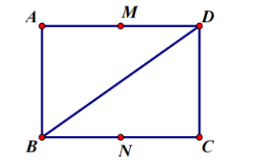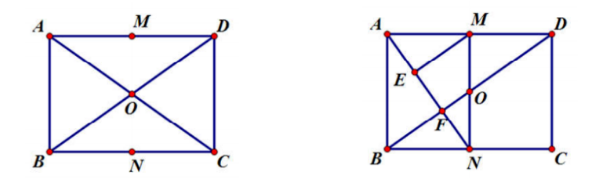$\sqrt{3} a ;\left(\frac{2 \sqrt{21}}{3} a, 2 \sqrt{3} a\right)$

#### 解析：

$M N$ 的距离 $d=\sqrt{O M^2-\left(\frac{M N}{2}\right)^2} \in\left(0, \frac{\sqrt{6}}{3} a\right)$,